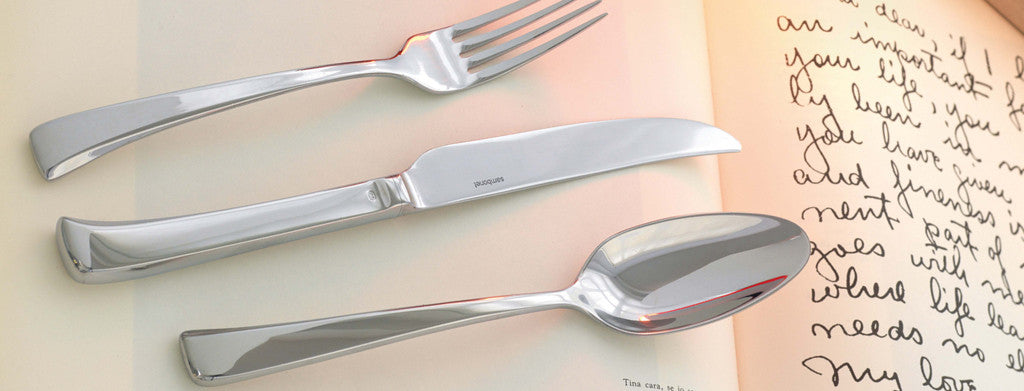Shopping Cart

### Refine

View all

#### Price# Cutlery£1.43 each (ex VAT)£15.16£0.55 each (ex VAT)£9.48 each (ex VAT)£9.48 each (ex VAT)£17.24£17.24£0.34 each (ex VAT)£0.55 each (ex VAT)£0.53 each (ex VAT)£0.52 each (ex VAT)£0.52 each (ex VAT)£1.43 each (ex VAT)£0.76 each (ex VAT)£1.30 each (ex VAT)£9.48 each (ex VAT)£1.63 each (ex VAT)£1.63 each (ex VAT)£19.82 each (ex VAT)£17.42£17.42£17.24 each (ex VAT)£19.01 each (ex VAT)£15.47 each (ex VAT)£16.59£16.59£0.55 each (ex VAT)£0.55 each (ex VAT)£0.53 each (ex VAT)£0.53 each (ex VAT)£0.53 each (ex VAT)£0.34 each (ex VAT)£0.34 each (ex VAT)£0.55 each (ex VAT)£0.46 each (ex VAT)£1.43 each (ex VAT)£1.61 each (ex VAT)£1.43 each (ex VAT)£0.73 each (ex VAT)£0.83 each (ex VAT)£1.43 each (ex VAT)£1.43 each (ex VAT)£1.31 each (ex VAT)£0.76 each (ex VAT)£0.75 each (ex VAT)£0.76 each (ex VAT)£0.45 each (ex VAT)£0.79 each (ex VAT)